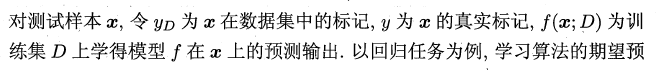#### 关于方差和偏差和噪声

统计/机器学习 监督式学习    浏览次数：2762        分享

0y_i 是第i行数据真实标签

y_pred_i 是第i行数据预测标签，mean_y_pred是所有预测值的均值a651021213   2018-10-13 14:27

##### 1个回答
0

$$\sum_{i=1}^n\frac{y_i-\hat y_i}{n}$$

$$\sum_{i=1}^n\frac{(\hat y_i-\text{mean}(\hat y))^2}{n-1}$$

SofaSofa数据科学社区DS面试题库 DS面经MangoCoke   2018-10-14 09:57相关主题

KNN中K值的选择   5回答